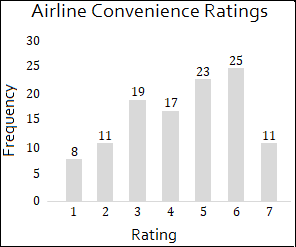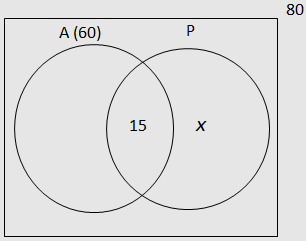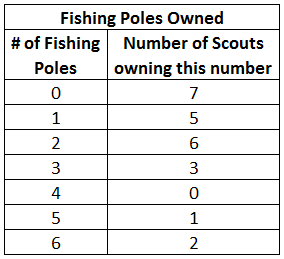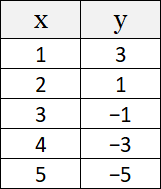# TSI Math Practice Test

TSI Math Test Prep

If you are serious about getting a great score on your TSI math test, try out our recommended TSI Math Prep Course.

Below is our free TSI Math Practice Test. These TSI Math questions are similar to those found on the Texas Success Initiative Assessment 2.0. Topics covered on this test are: (1) Quantitative Reasoning (2) Algebraic Reasoning (3) Geometric and Spatial Reasoning (4) Probabilistic and Statistical Reasoning. You may use a calculator.
Congratulations - you have completed . You scored %%SCORE%% out of %%TOTAL%%. Your performance has been rated as %%RATING%%
 Question 1

### Bill's new porch is rectangular, with an area of 50 square feet. If the length of the porch is two times the width, what is the perimeter of the porch?

 A 30 feet B 40 feet C 50 feet D 60 feet
Question 1 Explanation:
The correct answer is (A). The formula for the area of a rectangle:

$A = \text{Width} \cdot \text{Length}$

From the question we know that $L = 2W$ and that $A = 50$. So we can plug these values into our equation and solve for $W$:

$50 = W \cdot L$
$50 = W \cdot 2W$
$50 = 2W^2$
$W^2 = 25$
$W = 5$

We know that $L = 2W$, so $L = 10$
Perimeter $= 2L + 2W$
Perimeter $= 2 \cdot 10 + 2 \cdot 5$
Perimeter $= 30$
 Question 2

### What is the probability of selecting a male from a group of 4 males and 8 females?

 A $\dfrac{1}{3}$ B $\dfrac{1}{2}$ C $\dfrac{2}{3}$ D $\dfrac{4}{5}$
Question 2 Explanation:
The correct answer is (A). To find the probability of selecting a male, you need to divide the total number of males by the total number of people:

$P(\text{Male}) = \frac{\text{Number of Males}}{\text{Total Number of People}}$

$P(\text{Male}) = \frac{4}{12}$

$P(\text{Male}) = \frac{1}{3}$
 Question 3

### $3(x + 1) = 5(x − 2) + 7$

 A −2 B 2 C ½ D 3
Question 3 Explanation:
The correct answer is (D). We can simplify the equation as follows:

$3(x + 1) = 5(x − 2) + 7$
$3x + 3 = 5x − 10 + 7$
$3x + 3 = 5x − 3$

We can add $3$ to both sides to get the following:

$3x + 6 = 5x$

We can subtract $3x$ from both sides and then divide the resulting equation by $2$ to solve for $x$ as follows:

$6 = 2x$
$3 = x$
 Question 4

### Line A passes through the point $(−1, 2)$. Which of the following CANNOT be the equation of line A?

 A $y = 1 − x$ B $y = x + 1$ C $x = −1$ D $y = x + 3$
Question 4 Explanation:
The correct answer is (B). The slope-intercept form of a line is:

$y = mx + b$

Since the line passes through $(−1, 2)$, there are three possibilities: The line will have a slope (the “$m$” in front of the “$x$” variable), it will be vertical $(x = −1)$, or it will be horizontal $(y = 2)$.

Plug $x = −1$ into all four equations to see which equation is not satisfied. The only answer choice that doesn’t give us $y = 2\,$ is $\,y = x + 1$.
 Question 5

### Diego’s current age is five times Martina’s age ten years ago. If Martina is currently $m$ years old, what is Diego’s current age in terms of $m$?

 A $5m$ B $5m − 10$ C $5m − 50$ D $5m + (m − 10)$
Question 5 Explanation:
The correct answer is (C). Martina’s age ten years ago is:
$m − 10$

So Diego’s age is:

$5(m − 10)$
$= 5m − 50$
 Question 6

### If $2x + 5 = 8x$, then $12x = \; ?$

 A 5 B 10 C 15 D 20
Question 6 Explanation:
The correct answer is (B). Here is the easiest way to solve this:

$2x + 5 = 8x$
$8x − 2x = 5$
$6x = 5$
$6x \cdot 2 = 5 \cdot 2$
$12x = 10$
 Question 7

### An airline asked its agents to assign convenience ratings on a scale of 1–7 to 114 flights leaving one of its hub cities. The histogram below shows the distribution of the ratings of the 114 flights. What is the median rating assigned by the agents?A 3 B 4 C 5 D 6
Question 7 Explanation:
The correct answer is (C). The median is the value at the midpoint of a frequency distribution of observed values. Since we have an even number of ratings (114), the median will be the average of the two middle numbers. In this case, the median rating will be the average of the 57th and 58th rating.

Add the frequencies up, starting from the lowest rating:

Rating 1: Flights 1–8 (the first 8)
Rating 2: Flights 9–19 (the next 11)
Rating 3: Flights 20–38 (the next 19)
Rating 4: Flights 39–55 (the next 17)
Rating 5: Flights 56–78 (the next 23)
......

You could keep going, but you can see that both the 57th and the 58th rating will correspond to a rating of 5.

Therefore, the median rating is 5.
 Question 8

### $\sqrt{2x} = 4$

 A 1 B 4 C 8 D 16
Question 8 Explanation:
The correct answer is (C). We can solve for $x$ by first squaring both sides and solving as follows:

$\sqrt{2x} = 4$

$(\sqrt{2x})^2 = (4)^2$

$2x = 16$

$x = 8$
 Question 9

### The market price of a house is directly proportional to the total area of the house in square feet. If the price of a 1,500 square foot home is $300,000 what is the price of a 2,000 square foot home?  A$350,000 B $400,000 C$450,000 D $500,000 Question 9 Explanation: The correct answer is (B). We can solve this by setting up a proportion using the ratio of area to price:$\dfrac{1{,}500}{$300{,}000}= \dfrac{2{,}000}{x}$

Cross multiply and solve for $x$:

$1{,}500x = \$600{,}000{,}000x = \$400{,}000$
 Question 10

### $2y = 3x − 6$

 A −2 B −1 C 1 D 2
Question 10 Explanation:
The correct answer is (D). At the $x$-intercept, $y = 0$. We can solve for the $x$-intercept by setting $y = 0$ in the equation of the line:

$2y = 3x − 6$
$2(0) = 3x − 6$
$0 = 3x − 6$
$3x = 6$
$x = 2$
 Question 11

### There are 80 students in a school. Every student is taking either algebra or pre-calculus, and some students are taking both. There are 60 students in the algebra class. If 15 students are taking both algebra and pre-calculus, what is the probability that a randomly selected student is taking pre-calculus but not algebra?

 A $\dfrac{1}{4}$ B $\dfrac{1}{3}$ C $\dfrac{3}{8}$ D $\dfrac{3}{4}$
Question 11 Explanation:
The correct answer is (A). We can set up a Venn diagram to help us determine the number of students taking pre-calculus but not algebra:Students taking algebra but not pre-calculus $= 45$

We can figure out the number of students taking pre-calculus but not algebra $(x)$ as follows:

$45 + 15 + x = 80$
$x = 20$

Therefore, the probability that a student is taking pre-calculus but not algebra is:

$P(x) = \dfrac{20}{80} = \dfrac{1}{4}$
 Question 12

### $\dfrac{2}{3}x + 2 = 4$

 A 3 B 4 C 5 D 6
Question 12 Explanation:
The correct answer is (A). We can solve for $x$ by subtracting $2$ from both sides of the equation, then multiplying both sides by $3$, and finally dividing both sides by $2$ to get the value of $x$:

$\dfrac{2}{3}x + 2 = 4$

$\dfrac{2}{3}x = 2$

$2x = 6$

$x = 3$
 Question 13

### $x^2 + 8x + 15 = 0$

 A (2, 3) B (3, 5) C (−2, −3) D (−3, −5)
Question 13 Explanation:
The correct answer is (D). In order to factor the quadratic equation, we need to find the two factors of $15$ (the constant term) that sum to $8$ (the coefficient of $x$):

$15 = 3 \cdot 5$
$8 = 3 + 5$

So, $3$ and $5$ are the two factors that meet our requirement. The fully factored form of the quadratic is:

$(x + 3)(x + 5) = 0$

Recall that if $ab = 0$, then either $a = 0$, or $b = 0$.

If $(x + 3)(x + 5) = 0$
Then either $(x + 3) = 0$, or $(x + 5) = 0$
That is, either: $x = −3$, or $x = −5$
The roots of the quadratic are: $−3$, and $−5$
 Question 14

### $\sqrt{5}\sqrt{3} = \; ?$

 A $\sqrt{2}$ B $\sqrt{8}$ C $\sqrt{15}$ D $8$
Question 14 Explanation:
The correct answer is (C). We can use the following rule of radicals to answer this question:

$\sqrt{a} \sqrt{b} = \sqrt{a \cdot b}$

$\sqrt{5}\sqrt{3} = \sqrt{5 \cdot 3} = \sqrt{15}$
 Question 15

### The office supply superstore sells pens for 60 cents each and pencils for 40 cents each. Diane purchased a total of 17 items (pens and pencils) for \$8.20. How many pens did Diane purchase?  A 5 B 7 C 10 D 12 Question 15 Explanation: The correct answer is (B). The first step is to define the variables: Let the number of pens Diane purchased$= x$Let the number of pencils Diane purchased$= y$We can find the number of pens Diane purchased by writing two equations and solving for$x$as follows.$0.6x + 0.4y = 8.2x + y = 17y = 17 − x0.6x + 0.4(17 − x) = 8.20.6x + 6.8 − 0.4x = 8.20.2x = 1.4x = \dfrac{1.4}{0.2} = \dfrac{14}{2} = 7$ Question 16 ### A scoutmaster is preparing his troop for their annual fishing expedition. He surveys each of his scouts to find out how many fishing poles they own. What is the mode of the number of poles owned?A 0 B 1 C 2 D 3 Question 16 Explanation: The correct answer is (A). The mode of the data is the number that appears the most often. In this case the largest number of scouts (7) own 0 fishing poles.  Question 17 ### Simplify:$(x^6)(x^5)$ A$2x^{11}$B$2x^{30}$C$x^{11}$D$x^{30}$Question 17 Explanation: The correct answer is (C). When multiplying exponents with the same base, you should add the exponents:$(x^6)(x^5) = x^{5 + 6}= x^{11}$ Question 18 ### The points represented by the$(x, y)$coordinate pairs in the table below all lie on line$k$. What is the slope of line$k$?A −2 B −3 C 2 D 5 Question 18 Explanation: The correct answer is (A). We are told that all the points lie on line$k$. Therefore, we can calculate the slope as the change in$y$divided by the change in$x$using any two of the points. Using points$(1, 3)$and$(5, −5)$the slope is calculated as follows:$\text{Slope}= \dfrac{y_2 − y_1}{x_2 − x_1} = \dfrac{−5 − 3}{5 − 1}= \dfrac{−8}{4}= −2$ Question 19 ###$x^4 − 16 = \; ?$ A$(x + 2)(x − 2)(x^2 + 4)$B$(x + 2)^2(x − 2)^2$C$(x + 2)^3 (x − 2)^1$D$(x − 2)^4$Question 19 Explanation: The correct answer is (A). First we can rewrite the original expression:$x^4 − 16= (x^2)^2 − 4^2$We can simplify by using the identity:$a^2 − b^2 = (a − b)(a + b)$As follows:$(x^2)^2 − 4^2= (x^2 − 4)(x^2 + 4)= (x^2 − 2^2)(x^2 + 4)= (x − 2)(x + 2)(x^2 + 4)$ Question 20 ### What is the maximum value of this function? ###$f(x) = −16x^2 + 32x + 20$ A 32 B 34 C 36 D 40 Question 20 Explanation: The correct answer is (C). The graph of the given function is a parabola that opens downward, as indicated by the$−16$. There are several techniques for finding the maximum value of a quadratic function of the form$f(x) = ax^2 + bx + c$. The easiest way to solve this problem is to use the formula for finding the coordinates of the vertex of a parabola as follows (recall that the vertex of a downward facing parabola will be its maximum value): The vertex of a parabola is located at the point:$\left(−\dfrac{b}{2a}, \, c − \dfrac{b^2}{4a}\right)$In this problem:$a = −16, \, b = 32, \, c = 20$The$y$-coordinate of the vertex of the parabola will have a value of:$c − \dfrac{b^2}{4a}= 20 − \dfrac{32^2}{4(−16)}= 20 + 16 = 36\$
Once you are finished, click the button below. Any items you have not completed will be marked incorrect.
There are 20 questions to complete.
 ← List →